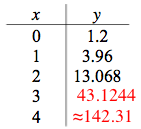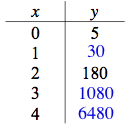### Home > CCA > Chapter 7 > Lesson 7.1.2 > Problem7-24

7-24.

Each table below represents an exponential function of the form $y=ab^x$. Copy and complete each table on your paper and find the corresponding equation. Homework Help ✎

1.  x y 0 1.2 1 3.96 2 13.068 3 4

What is the multiplier?y = 1.2(3.3)x

1.  x y 0 5 1 2 180 3 4y = 5(6)x